Name:    mpm1D - 6.4 - Parallel Perp 2

Multiple Choice
Identify the choice that best completes the statement or answers the question.

1.

The lines in which pair are perpendicular?
 a.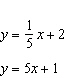b.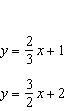c.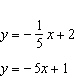d.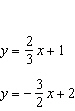2.

What is the slope of the line that is perpendicular to the line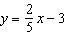?
 a.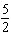c.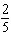b.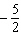d.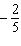3.

What is the x-intercept for the line y = 3x – 6?
 a. 0 c. 3 b. 2 d. –6

4.

Which statement is NOT true for the properties of the line?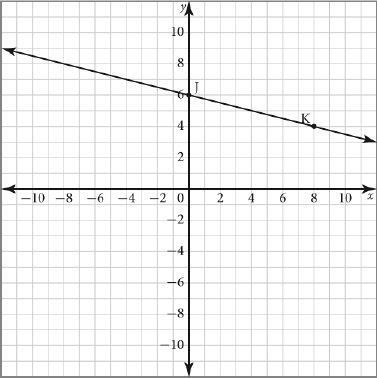a. The y-intercept is 6. c. The run from point J to point K is 8. b. The slope of the line is negative. d. The rise from point J to point K is 2.

5.

What is the equation of the line containing points M and N?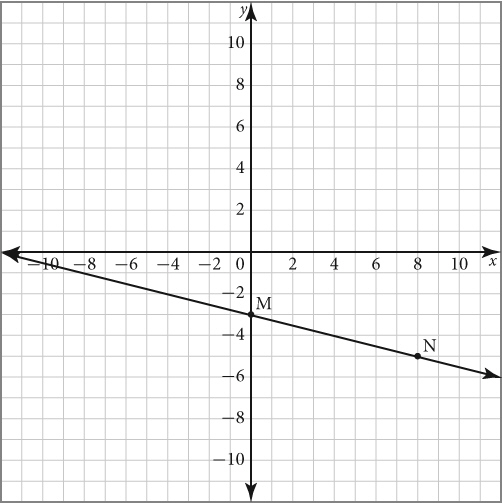a. y =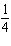x – 3 c. y = 4x – 3 b. y =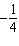x – 3 d. y = –4x – 3

6.

The equation of line A is y = 4x – 8. Which equation represents a line that is parallel to line A?
 a. y = –4x – 8 c. y = 4x + 8 b. y = 8x – 4 d. y = –8x + 4

7.

The equation of the line containing LM is y = 0.5x + 4. The two lines are parallel. Find the equation of the line containing NP.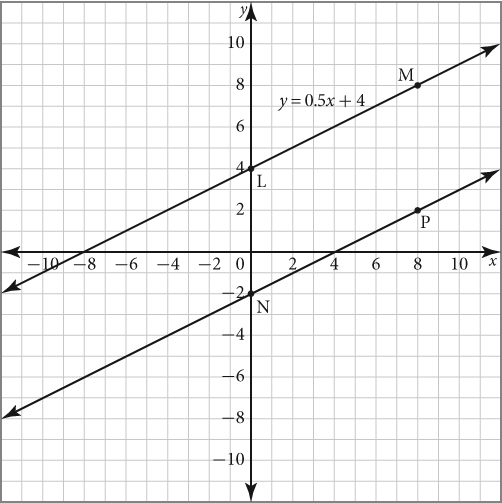a. y = 0.5x + 2 c. y = 2x + 2 b. y = 0.5x – 2 d. y = 2x – 2

8.

Which statement is NOT true about the properties of lines 1 and 2?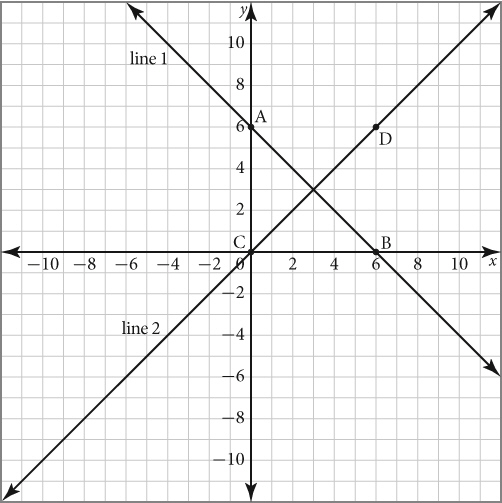a. Line 1 has negative slope. b. Line 2 has positive slope. c. The y-intercept of line 1 is greater than the y-intercept of line 2. d. Line 1 and Line 2 are not perpendicular